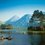# OPC 2 Problem 2

$2. \text{Prove that no number in this sequence } 11,111,1111,11111,........... \\ \text{ is a perfect square}$

Give different methods of solving this. This was a problem to the OPC 2. The OPC 2 has already ended.Note by Rajdeep Dhingra
6 years, 2 months ago

This discussion board is a place to discuss our Daily Challenges and the math and science related to those challenges. Explanations are more than just a solution — they should explain the steps and thinking strategies that you used to obtain the solution. Comments should further the discussion of math and science.

When posting on Brilliant:

• Use the emojis to react to an explanation, whether you're congratulating a job well done , or just really confused .
• Ask specific questions about the challenge or the steps in somebody's explanation. Well-posed questions can add a lot to the discussion, but posting "I don't understand!" doesn't help anyone.
• Try to contribute something new to the discussion, whether it is an extension, generalization or other idea related to the challenge.
• Stay on topic — we're all here to learn more about math and science, not to hear about your favorite get-rich-quick scheme or current world events.

MarkdownAppears as
*italics* or _italics_ italics
**bold** or __bold__ bold
- bulleted- list
• bulleted
• list
1. numbered2. list
1. numbered
2. list
Note: you must add a full line of space before and after lists for them to show up correctly
paragraph 1paragraph 2

paragraph 1

paragraph 2

[example link](https://brilliant.org)example link
> This is a quote
This is a quote
    # I indented these lines
# 4 spaces, and now they show
# up as a code block.

print "hello world"
# I indented these lines
# 4 spaces, and now they show
# up as a code block.

print "hello world"
MathAppears as
Remember to wrap math in $$ ... $$ or $ ... $ to ensure proper formatting.
2 \times 3 $2 \times 3$
2^{34} $2^{34}$
a_{i-1} $a_{i-1}$
\frac{2}{3} $\frac{2}{3}$
\sqrt{2} $\sqrt{2}$
\sum_{i=1}^3 $\sum_{i=1}^3$
\sin \theta $\sin \theta$
\boxed{123} $\boxed{123}$

## Comments

Sort by:

Top Newest

Don't get me wrong, but I feel like most of the problems are from "an excursion in mathematics"

- 6 years, 2 months ago

Log in to reply

Well I have heard a lot about that book. But these problems I have thought up and one is a famous one from IMO.

- 6 years, 2 months ago

Log in to reply

OK Allright :)

- 6 years, 2 months ago

Log in to reply

write

$\underbrace {1 \cdots 1} _ {n \textrm{ times}}= a^2$

Of course $a$ must be odd (and greater than $1$ since our sequence begins with $11$, in other words $n \geq 2$ ). Consider the even $b = a -1$ . We have

$\underbrace {1 \cdots 1} _ {n \textrm{ times}}= b^2 -2b +1$

$\underbrace {1 \cdots 1} _ {n-1 \textrm{ times}}\times 10= b^2 - 2b$

$b$ is even so $4$ divides the LHS, but clearly not th RHS. Contradiction.

- 6 years, 2 months ago

Log in to reply

The numbers in the sequence can be written as $111....108+3$ now since the last two digits, i.e. $08$, is divisible by $4$, the numbers are of the form of $4k+3$. Now note that a perfect square, say $a^2$ when written in modulo $4$$\implies a^2=0,1,2\pmod4$ but not $=3\pmod4$ which contradicts to the form of the numbers in the sequence.

- 6 years, 2 months ago

Log in to reply

any no will have last two digits 11 and therefore cannot be a perfect square .i hope u get it .if u dont just ask

- 5 years, 5 months ago

Log in to reply

You mean any number with last 2 digits 11, 31, 51, 71, 91 cannot be a perfect square?

- 5 years, 5 months ago

Log in to reply

Yup, It can't be.

- 5 years, 5 months ago

Log in to reply

absolutely.

- 5 years, 5 months ago

Log in to reply

×

Problem Loading...

Note Loading...

Set Loading...